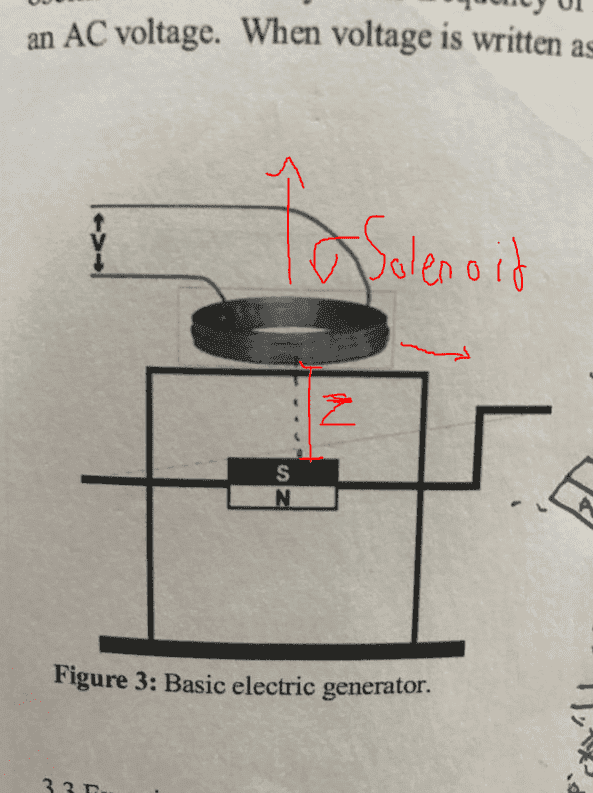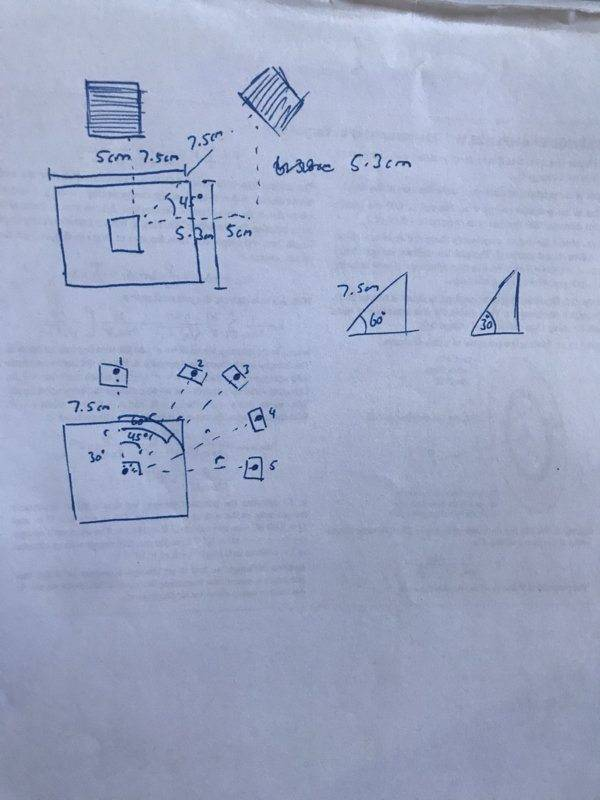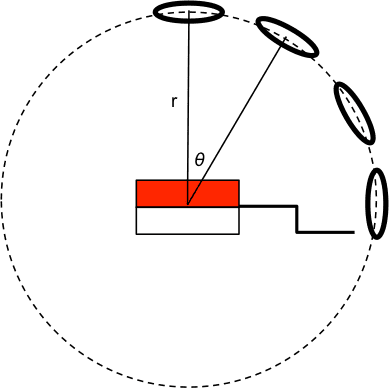# EMF induced in an off-axis solenoid due to a rotating magnet

Cactus
Homework Statement:
Hey, I have come up with an idea for my EEI to measure the emf induced in a solenoid by a rotating magnet when the solenoid is not directly above the magnet (such that the magnetic field is not uniform). Previously I had to perform an experiment where the solenoid was on axis (see picture) and now I plan to further the experiment by moving it up and down (increasing height from the magnet) and left and right (increasing horizontal distance from the magnet) and I was wondering if anyone could point me in the right direction for deriving a formula for the off axis emf. Currently below are the two formulas for the on axis emf.

In the experiment it will be possible to measure the emf and thus the V and then calculate the field strength at that point (As N, w and A are constant). This field strength can then be used to determine the magnetic dipole moment, however it could also be used to check what factors affect the strength. So far I'm guessing the off axis magnet will only be affected by distance from the magnet, and thus only the z factor in the final formula will affect the field strength and this could be tested by measuring the dipole and using theoretical z values to find field strength, and comparing this to experimental values of field strength (However I cant check this until I perform the experiment this week).

But apart from that I could imagine the angle between the solenoid and the magnet having an effect, however I can't see how I would derive a theoretical formula for it at this point in time

Any help would be appreciated
Thanks!
Relevant Equations:
emf = -wNBAsin(wt)
Where emf is induced in solenoid
w is angular frequency
N is number of turns in the solenoid
B is the magnetic field strength
A is the area of the solenoid

V = wNBA
V is the voltage in the solenoid
w, N, B, A as before

B = u/2pi * 2*U / z^3
B is field strength
u is constant 1.26*10^-6
z is the distance from the centre of the magnet along the axis
U is the magnetic dipole momentThe red arrows correspond to the areas the solenoid will be moved too

•Delta2

Homework Helper
Gold Member
The formula you used for the induced voltage in your previous experiment is only an approximation of the actual result. It was obtained on the assumption that the B field due to the permanent magnet is uniform over the surface of the pick-up coil (I prefer that name to solenoid) and points in the ##+z##-direction, perpendicular to the plane of the coil. This assumption works for ##z >>R## where ##R## is the coil radius. If you move the coil away from the ##z##-axis, I suggest that you not move it parallel to itself because the dependence of the B-field on the radial direction becomes unnecessarily complicated. Instead, consider turning the coil as you move it so that its plane is always perpendicular to the radius ##r## from the center of the magnet to the center of the coil. Then plot the induced rms voltage as a function of spherical angle ##\theta## from the ##z##-axis. The coil must be in the ##xz## plane. In that case, the dipolar approximation for the magnetic field that you have already used predicts that the voltage as a function of angle at fixed radius ##r## varies according to ##V(\theta)=V(0)\cos\theta##, where ##V(0)## is the rms voltage at zero angle that you have already investigated.

Last edited:
Homework Helper
Gold Member
[...] ##V(\theta)=V(0)\cos\theta##, where ##V(0)## is the rms voltage at zero angle that you have already investigated.
Not to be nitpicky, but I think you mean "peak" voltage (rather than rms voltage).•kuruman
Homework Helper
Gold Member
Not to be nitpicky, but I think you mean "peak" voltage (rather than rms voltage).Thanks. Message edited to convey that ##V(0)## is whatever is measured at zero angle, peak or rms. I had rms because that's what one usually measures with a voltmeter.

•collinsmark
Cactus
The formula you used for the induced voltage in your previous experiment is only an approximation of the actual result. It was obtained on the assumption that the B field due to the permanent magnet is uniform over the surface of the pick-up coil (I prefer that name to solenoid) and points in the ##+z##-direction, perpendicular to the plane of the coil. This assumption works for ##z >>R## where ##R## is the coil radius. If you move the coil away from the ##z##-axis, I suggest that you not move it parallel to itself because the dependence of the B-field on the radial direction becomes unnecessarily complicated. Instead, consider turning the coil as you move it so that its plane is always perpendicular to the radius ##r## from the center of the magnet to the center of the coil. Then plot the induced rms voltage as a function of spherical angle ##\theta## from the ##z##-axis. The coil must be in the ##xz## plane. In that case, the dipolar approximation for the magnetic field that you have already used predicts that the voltage as a function of angle at fixed radius ##r## varies according to ##V(\theta)=V(0)\cos\theta##, where ##V(0)## is the rms voltage at zero angle that you have already investigated.

Hey, thanks for the reply and insight
I was just wondering when you explaining you're above statement would you mean something like thiswhere say for the top example if the magnet housing was 5 x 5cm (From memory I think it is) and I wanted to measure at a distance r = 7.5cm, I would move the magnet around keeping it perpendicular with the dipole moment of the magnet and keeping it a distance of 7.5cm, so that for an angle of 45 from the x (also then 45 from the z) it would be 7.5sin45 and 7.5cos45 on the x and y coordinates respectively. And then say for the second sketch at the bottom it would be some combination like that, where the angle in your formula you've given corresponds to that angle 30, 45, 60 and 90 from the z (Where the expected value for 90 would be zero, but because the field is not uniform it will be slightly higher experimentally)?

Also, in the case that I'm told I can't perform this experiment, for the original experiment (where the magnet is moved parallel along the x axis) would the calculation/formula be something derivable by hand or would it just be too complicated for that?
Thanks again!